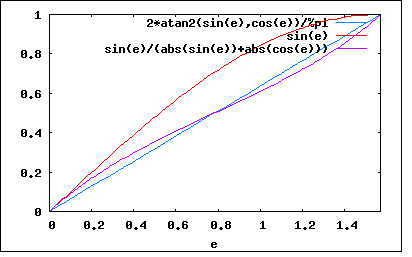# File:AngleApproximationQuality.png

AngleApproximationQuality.png(412 × 257 pixels, file size: 4 KB, MIME type: image/png)

Graph showing that $$\frac{y}{|x|+|y|}$$ gives a (much) better estimate of the angle than a sine ($$\frac{y}{\sqrt{x^2+y^2}}$$). Note that the blue line is the ideal line.

## File history

Click on a date/time to view the file as it appeared at that time.

Date/TimeThumbnailDimensionsUserComment
current12:05, 17 April 2009412 × 257 (4 KB)Jaspervdg (talk | contribs)Graph showing that $\frac{y}{|x|+|y|}$ gives a (much) better estimate of the angle than a sine ($\frac{y}{\sqrt{x^2+y^2}}$).
• You cannot overwrite this file.

The following page uses this file: